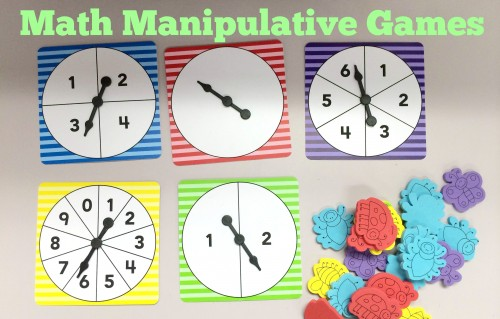# Math Manipulative GamesMany teachers know that manipulatives are helpful for teaching math to kids, especially younger kids. Using physical objects is a hands-on approach to learning math that helps kids develop math concepts. Pairing number spinners with counters is a great way to teach math by allowing kids to problem solve and count. Here are some great math games to play using math manipulatives and number spinners.

As for adults, casino developers have developed a way to keep them entertained, and that is an online casino. It’s an effective way to keep their minds active while also making money when they win.Practice basic addition and subtraction facts by having students spin a number spinner, this will be the first value. Then have the students spin again on another number spinner, this is the second value.  Have the students subtract the second number from the first. Have the students state their answers and show them using counters.

Here’s another game you can play using number spinners:

Number Sense and Place Value Game for  2nd – 3rd Grade

Have students practice spinning any of the number spinners and then call out the number they land on. Students can write the number down in word form, to practice the correlation between the word and the numeral representation.

Have students practice this with larger numbers, asking them to spin twice or even three times to make two-and-three-digit numbers. For example, if a student spins three times, and first spins a 3, then a 6, then a 9, he or she should write down the number 369.

Then have them write “three hundred sixty-nine” next to the numerals. Have students practice comparing different numbers they spin. If they first spin the above number (369), then an 8, a 2, and a 1, student should write: 369<821.